# High School Math : How to find the surface area of a pyramid

## Example Questions

### Example Question #1 : How To Find The Surface Area Of A Pyramid

What is the surface are of a pyramid with a square base length of 15 and a slant height (the height from the midpoint of one of the side lengths to the top of the pyramid) of 12?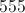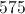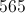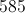Explanation:

To find the surface area of a pyramid we must add the areas of all five of the shapes creating the pyramid together.

We have four triangles that all have the same area and a square that supports the pyramid.

To find the area of the square we take the side length of 15 and square it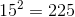The area of the square is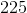.

To find the area of the triangle we must use the equation for the area of a triangle which is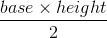Plug in the slant height 12 as the height of the triangle and use the side length of the square 15 as the base in our equation to get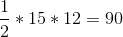The area of each triangle is.

We then multiply the area of each triangle by 4 to find the area of all four triangles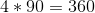.

The four triangles have a surface area of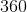.

We add the surface area of the four triangles with the area of the square to get the answer for the surface area of the pyramid which is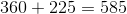.

The answer is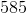.

### Example Question #2 : How To Find The Surface Area Of A Pyramid

Find the surface area of the following pyramid.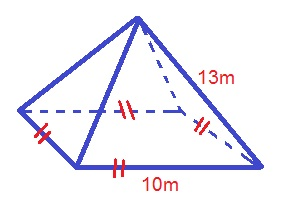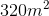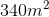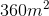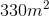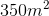Explanation:

The formula for the surface area of a pyramid is: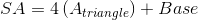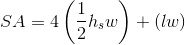Where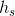is the length of the slant height,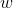is the width of the base, andis the length of the base

In order to determine the areas of the triangle, you will need to use the Pythagorean Theorem to find the slant height: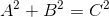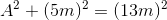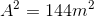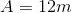Plugging in our values, we get: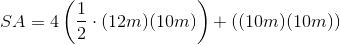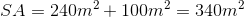### Example Question #3 : How To Find The Surface Area Of A Pyramid

Find the surface area of the following pyramid.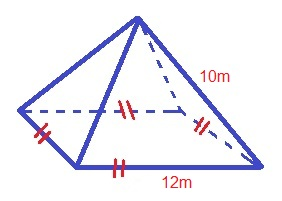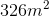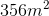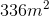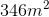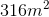Explanation:

The formula for the surface area of a pyramid is: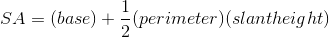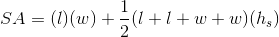Whereis the length of the base,is the width of the base, andis the slant height

Use the Pythagorean Theorem to find the length of the slant height: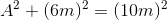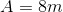Plugging in our values, we get: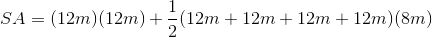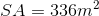### Example Question #4 : How To Find The Surface Area Of A Pyramid

What is the surface area of a square pyramid with a base side equal to 4 and a slant length equal to 6?Explanation:

The surface area of of a square pyramid can be determined using the following equation: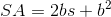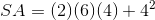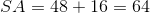### Example Question #5 : How To Find The Surface Area Of A Pyramid

What is the surface area of a square pyramid with a height of 12 in and a base side length of 10 in?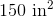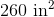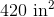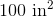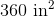Explanation:

The surface area of a square pyramid can be broken into the area of the square base and the areas of the four triangluar sides. The area of a square is given by: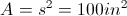The area of a triangle is: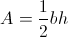The given height of 12 in is from the vertex to the center of the base. We need to calculate the slant height of the triangular face by using the Pythagorean Theorem: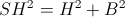where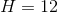and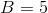(half the base side) resulting in a slant height of 13 in.

So, the area of the triangle is: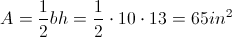There are four triangular sides totaling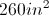for the sides.

The total surface area is thus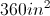, including all four sides and the base.